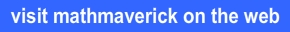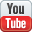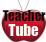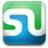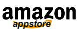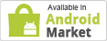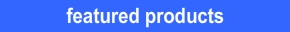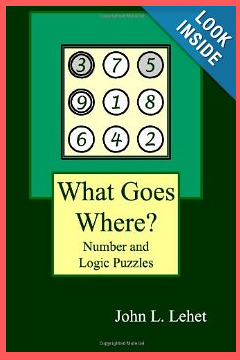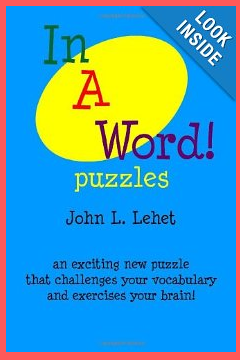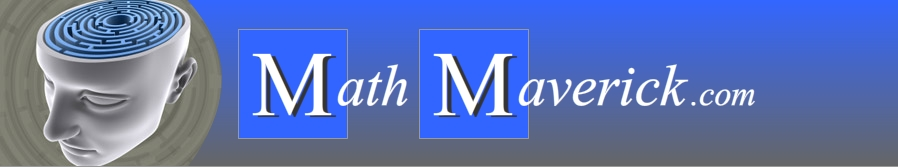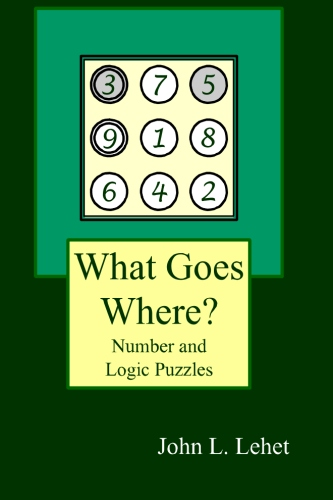Sneak Peak
Example Puzzle and Book Introduction

What Goes Where
Number and Logic Puzzles

 What Goes Where? Puzzles are a great introduction to problem solving. Using "hints", you have to correctly place numbers into a figure. In order to solve each puzzle, you must be able to follow simple directions, using reasoning skills to draw basic conclusions, understand basic number concepts and relationships and mentally perform basic arithmetic skills. Along the way to solving the puzzles, you will improve both your deductive reasoning and problem solving skills!
 purchase on Amazon.com for \$7.95 purchase on Kindle Direct for \$4.99